# announcements Web Studies test and Tutorial Tut Test

• Slides: 16
Download presentationannouncements Web. Studies test and Tutorial Tut Test 5 (formative assessment): Friday, 12 th 12: 00 → Tuesday, 16 th 18: 00 Tutorial 6: Thursday 18 th 14: 00the mole reading assignment: chapter 2 videos Web. Studies defining the molar mass amount-mass-number conversions mass percentage determine formula of unknown compound empirical/molecular formulas writing & balancing chemical equations calculating quantities (stoichiometry) mole-mole conversions from equations limiting reactants reaction yields fundamentals: solution stoichiometry molarity amount-mass-number conversions diluting molar solutions reactions in solutionlearning peer-assisted group learning (PAGL) interactive –style teaching enquiry based learning opportunities prepare for contact session: you do assigned reading you watch video clips Blackboard you work related sample problems text book/web you reflect: wonder, think about the concepts during contact session: we ask & test understanding of concepts we put up questions and discuss the content your group discusses/decides a response your scribe (guy in the middle) communicates your group compares group behind/front no-one calls answers, chews, misbehaves, etc.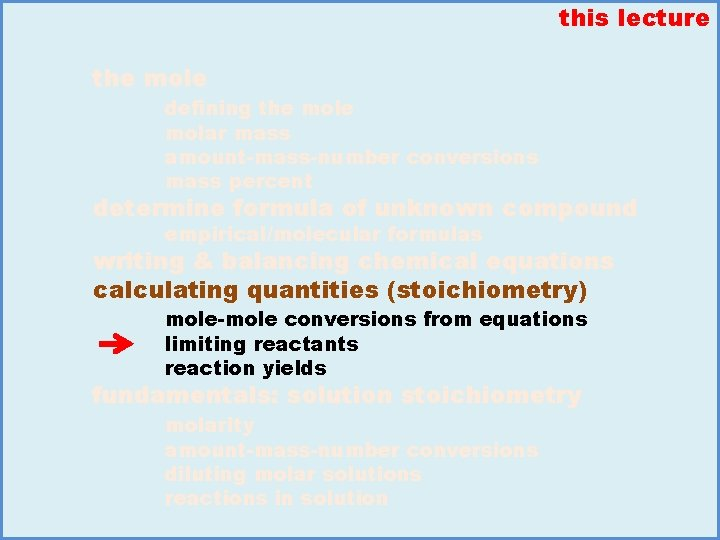this lecture the mole defining the molar mass amount-mass-number conversions mass percent determine formula of unknown compound empirical/molecular formulas writing & balancing chemical equations calculating quantities (stoichiometry) mole-mole conversions from equations limiting reactants reaction yields fundamentals: solution stoichiometry molarity amount-mass-number conversions diluting molar solutions reactions in solution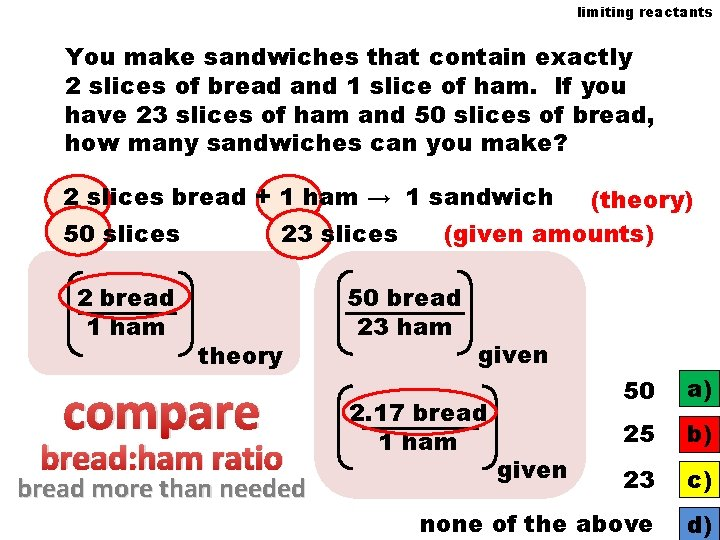limiting reactants You make sandwiches that contain exactly 2 slices of bread and 1 slice of ham. If you have 23 slices of ham and 50 slices of bread, how many sandwiches can you make? 2 slices bread + 1 ham → 1 sandwich 50 slices 2 bread 1 ham 23 slices theory compare bread: ham ratio bread more than needed (theory) (given amounts) 50 bread 23 ham given 50 a) 25 b) 23 c) none of the above d) 2. 17 bread 1 ham givenlimiting reactants You want to make sandwiches that specifically contain 2 slices of bread, 1 slice of ham and 3 slices of pickles. How many sandwiches can you make if you have 31 slices of bread, 17 slices of ham and 42 slices of pickles? theory limiting 2 bread + 1 ham + 3 pickles → 1 sandwich given amounts 31 17 42 1 sandwich = 15. 5 sandwich 31 bread × 2 bread 14 1 sandwich = 17 17 ham × 1 ham 15 1 sandwich = 14 42 pickles × 3 pickles 17 none of the above a) b) c) d)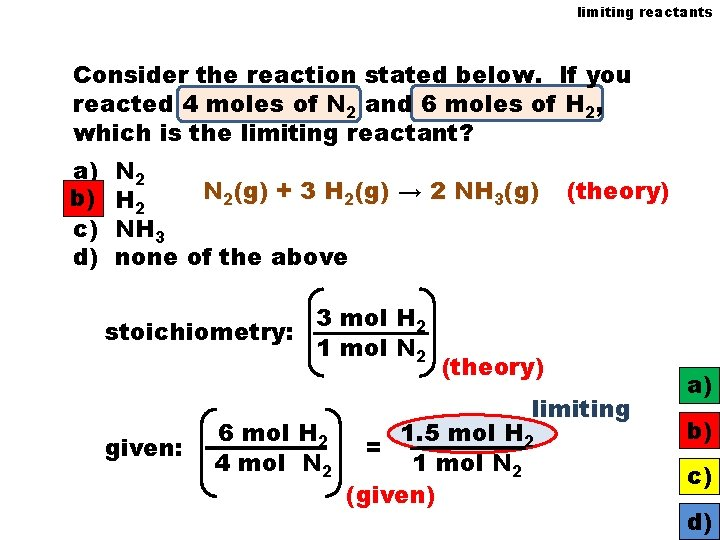limiting reactants Consider the reaction stated below. If you reacted 4 moles of N 2 and 6 moles of H 2, which is the limiting reactant? a) b) b) c) d) N 2(g) + 3 H 2(g) → 2 NH 3(g) H 2 NH 3 none of the above 3 mol H 2 stoichiometry: 1 mol N 2 given: 6 mol H 2 4 mol N 2 (theory) limiting 1. 5 mol H 2 = 1 mol N 2 (given) a) b) c) d)limiting reactants A reaction vessel contains 6 moles of N 2 and 4 moles of H 2 that reacts according to the reaction stated below. Which is the limiting reactant? N 2 + 3 H 2 → 2 NH 3 (mol): = 12 mol NH 3 3 H 2 1 N 2 limiting =4 2. 7 0. 6 HH 2 2 mol NH 3 1 N 2 6 theory given OR N 2 a) H 2 b) NH 3 c)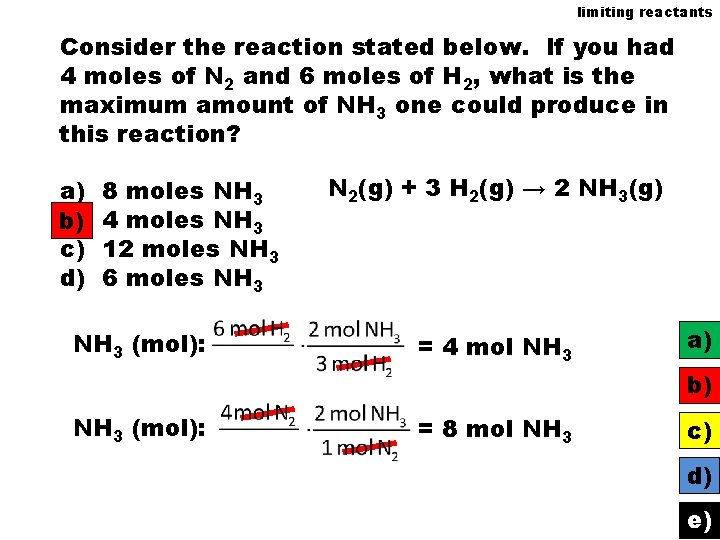limiting reactants Consider the reaction stated below. If you had 4 moles of N 2 and 6 moles of H 2, what is the maximum amount of NH 3 one could produce in this reaction? a) b) c) d) 8 moles NH 3 4 moles NH 3 12 moles NH 3 6 moles NH 3 (mol): N 2(g) + 3 H 2(g) → 2 NH 3(g) = 4 mol NH 3 a) b) NH 3 (mol): = 8 mol NH 3 c) d) e)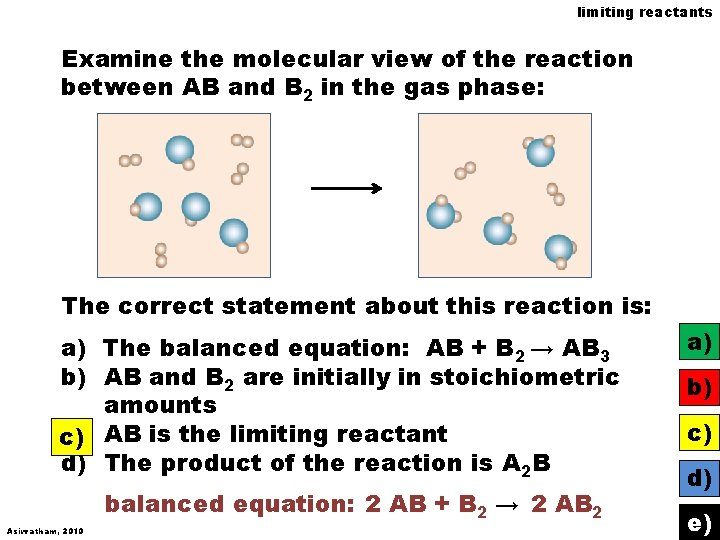limiting reactants Examine the molecular view of the reaction between AB and B 2 in the gas phase: The correct statement about this reaction is: a) The balanced equation: AB + B 2 → AB 3 b) AB and B 2 are initially in stoichiometric amounts c) AB is the limiting reactant d) The product of the reaction is A 2 B balanced equation: 2 AB + B 2 → 2 AB 2 Asirvatham, 2010 a) b) c) d) e)Identify whether this presentation represents the combustion of methane (CH 4) with c) limiting excess a) stoichiometric amount of oxygen b) methane limiting c) oxygen limiting CH 4 + 2 O 2 → CO 2 + 2 H 2 O stoichiometry: given: Wood, 2006 2 mole O 2 = 1 mol CH 4 2 mole O 2 5 mol CH 4 2 want 1 a) have b) 0. 4 = 1 c)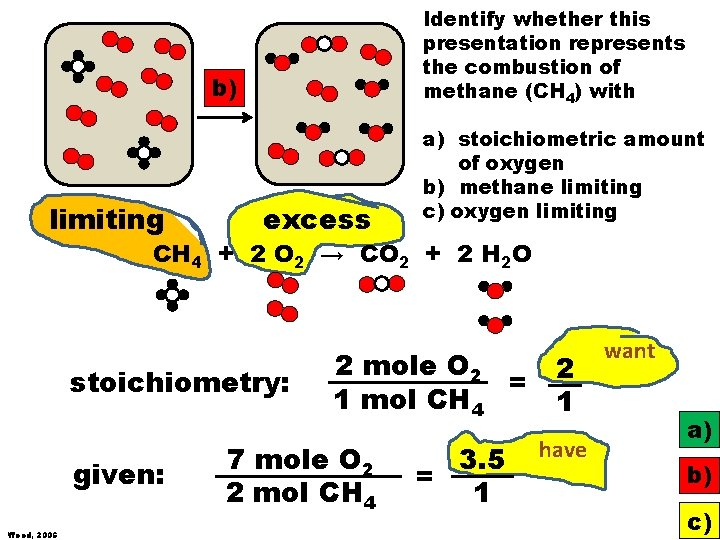Identify whether this presentation represents the combustion of methane (CH 4) with b) limiting excess a) stoichiometric amount of oxygen b) methane limiting c) oxygen limiting CH 4 + 2 O 2 → CO 2 + 2 H 2 O stoichiometry: given: Wood, 2006 2 mole O 2 = 1 mol CH 4 7 mole O 2 2 mol CH 4 3. 5 = 1 2 1 have want a) b) c)Identify whether this presentation represents the combustion of methane (CH 4) with a) a) stoichiometric amount of oxygen b) methane limiting c) oxygen limiting CH 4 + 2 O 2 → CO 2 + 2 H 2 O stoichiometry: given: Wood, 2006 2 mole O 2 = 1 mol CH 4 6 mole O 2 3 mol CH 4 2 = 1 2 1 have want a) b) c)limiting reactants 2 SO 2(g) + O 2(g) → 2 SO 3(g) Given 3. 0 moles of SO 2. O 2 would be the limiting reactant if we had less than 1. 5 ___ mol O 2 Given 5 moles of O 2. SO 2 would be the limiting 10 mol SO 2 reactant if we had less than ___ Given 3. 5 moles of O 2. SO 2 is in excess if we had more than 7. 0 ___ mol SO 2 Given 1. 5 moles of SO 2. O 2 is in excess if we had more than 0. 75 ____ mol O 2 0. 75 a) 1. 5 b) 10 c) 7. 0 d) 3. 5 e)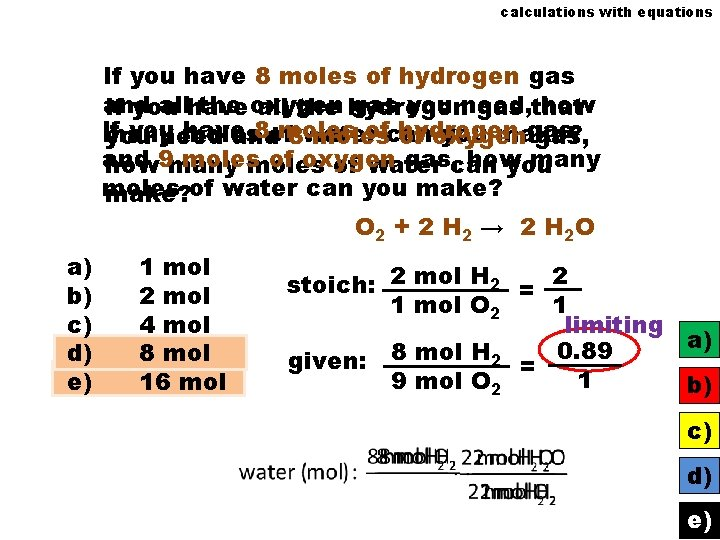calculations with equations If you have 8 moles of hydrogen gas and allhave the oxygen gas you need, how If you all the hydrogen gas that If you have 8 of moles ofcan hydrogen gas many moles you make? you need and 8 water moles of oxygen gas, and moles of oxygen gas, can how many how 9 many moles of water you moles make? of water can you make? O 2 + 2 H 2 → 2 H 2 O a) b) c) d) e) 1 mol 2 mol 4 mol 8 mol 16 mol stoich: 2 mol H 2 = 2 1 mol O 2 1 limiting a) 0. 89 given: 8 mol H 2 = 1 9 mol O 2 b) c) d) e)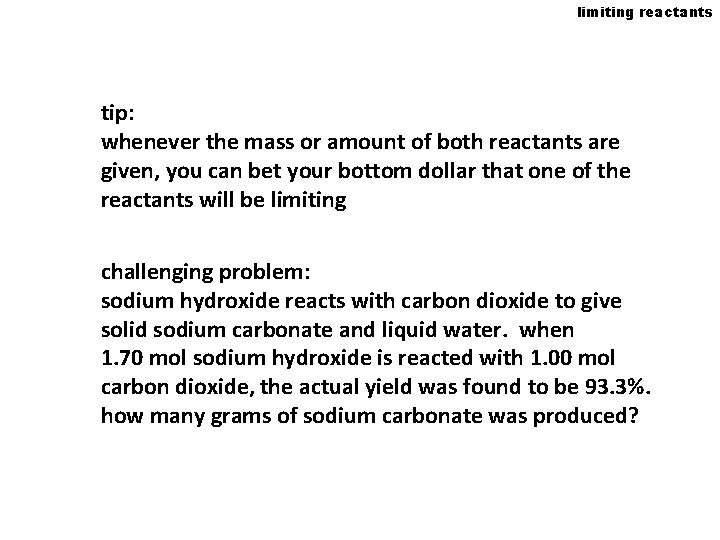limiting reactants tip: whenever the mass or amount of both reactants are given, you can bet your bottom dollar that one of the reactants will be limiting challenging problem: sodium hydroxide reacts with carbon dioxide to give solid sodium carbonate and liquid water. when 1. 70 mol sodium hydroxide is reacted with 1. 00 mol carbon dioxide, the actual yield was found to be 93. 3%. how many grams of sodium carbonate was produced?